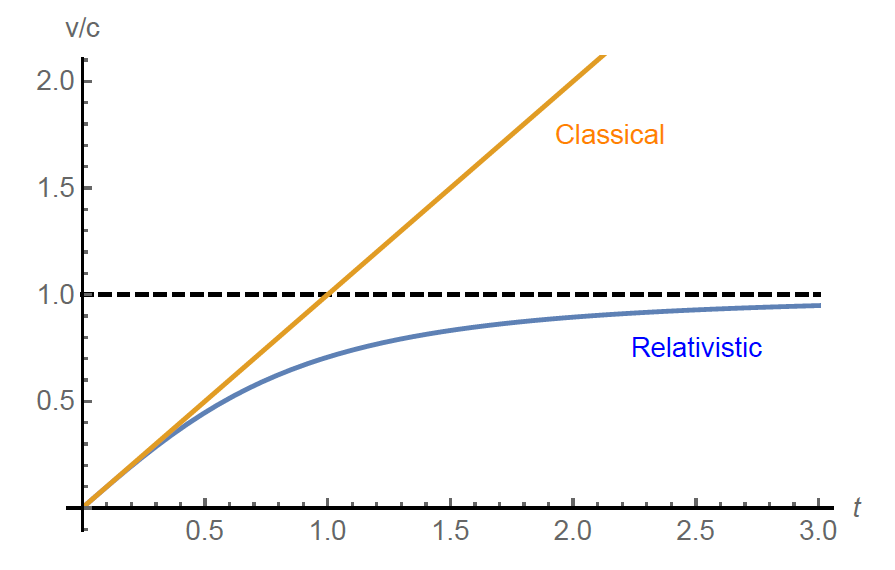# 15.2: The Four-Acceleration

$$\newcommand{\vecs}{\overset { \rightharpoonup} {\mathbf{#1}} }$$ $$\newcommand{\vecd}{\overset{-\!-\!\rightharpoonup}{\vphantom{a}\smash {#1}}}$$$$\newcommand{\id}{\mathrm{id}}$$ $$\newcommand{\Span}{\mathrm{span}}$$ $$\newcommand{\kernel}{\mathrm{null}\,}$$ $$\newcommand{\range}{\mathrm{range}\,}$$ $$\newcommand{\RealPart}{\mathrm{Re}}$$ $$\newcommand{\ImaginaryPart}{\mathrm{Im}}$$ $$\newcommand{\Argument}{\mathrm{Arg}}$$ $$\newcommand{\norm}{\| #1 \|}$$ $$\newcommand{\inner}{\langle #1, #2 \rangle}$$ $$\newcommand{\Span}{\mathrm{span}}$$ $$\newcommand{\id}{\mathrm{id}}$$ $$\newcommand{\Span}{\mathrm{span}}$$ $$\newcommand{\kernel}{\mathrm{null}\,}$$ $$\newcommand{\range}{\mathrm{range}\,}$$ $$\newcommand{\RealPart}{\mathrm{Re}}$$ $$\newcommand{\ImaginaryPart}{\mathrm{Im}}$$ $$\newcommand{\Argument}{\mathrm{Arg}}$$ $$\newcommand{\norm}{\| #1 \|}$$ $$\newcommand{\inner}{\langle #1, #2 \rangle}$$ $$\newcommand{\Span}{\mathrm{span}}$$$$\newcommand{\AA}{\unicode[.8,0]{x212B}}$$

We can of course also define a four-vector version of the acceleration, by taking the derivative of the four-velocity with respect to the proper time. As with the forces, we’ll see that we’re in for some nasty surprises, this time because the proper time derivative acts on the $$\gamma(u)$$ factor in the velocity as well as on the components:

$\overline{\boldsymbol{a}} \equiv \frac{\mathrm{d} \overline{\boldsymbol{v}}}{\mathrm{d} \tau}=\gamma(v) \frac{\mathrm{d}}{\mathrm{d} t} \gamma(v)\left(c, v_{x}, v_{y}, v_{z}\right)=\left(\gamma^{4}(v) \frac{\boldsymbol{a} \cdot \boldsymbol{v}}{c}, \gamma^{2}(v) \boldsymbol{a}+\gamma^{4}(v) \frac{\boldsymbol{a} \cdot \boldsymbol{v}}{c^{2}} \boldsymbol{v}\right) \label{15.2.1}$

where we used the time derivative of the $$\gamma(u)$$ function

$\frac{\mathrm{d} \gamma}{\mathrm{d} t}=\frac{\mathrm{d}}{\mathrm{d} t} \frac{1}{\sqrt{1-(v / c)^{2}}}=-\frac{1}{2} \frac{1}{\left(1-(v / c)^{2}\right)^{3 / 2}} \cdot\left(-2 \frac{\boldsymbol{v}}{c^{2}} \cdot \frac{\mathrm{d} \boldsymbol{v}}{\mathrm{d} t}\right)=\gamma^{3}(v) \frac{\boldsymbol{v} \cdot \boldsymbol{a}}{c^{2}} \label{15.2.2}$

and we’ve introduced the (classical) acceleration three-vector as the coordinate time derivative of the velocity three-vector: $$\boldsymbol{a}=\mathrm{d} \boldsymbol{v} / \mathrm{d} t$$. As you can see in equation (\ref{15.2.1}), the four-acceleration has terms that scale with $$\gamma^{2}$$ and terms that scale with $$\gamma^{4}$$, making it an inconvenient object to work with. Geometrically though, it has a clean interpretation, which comes into view once you consider the inner product between the acceleration and velocity four-vectors:

\begin{aligned} \overline{\boldsymbol{a}} \cdot \overline{\boldsymbol{v}} &=\gamma^{5}(v)(\boldsymbol{a} \cdot \boldsymbol{v})-\gamma^{3}(v)(\boldsymbol{a} \cdot \boldsymbol{v})-\gamma^{5}(v) \frac{\boldsymbol{a} \cdot \boldsymbol{v}}{c^{2}}(\boldsymbol{v} \cdot \boldsymbol{v}) \\ &=\gamma^{3}(v)(\boldsymbol{a} \cdot \boldsymbol{v})\left[\frac{1-v^{2} / c^{2}}{1-v^{2} / c^{2}}-1\right] \\ &=0 \end{aligned}\label{15.2.3}

These four-vectors are therefore (in the four-vector sense) always perpendicular! That seems odd from a classical point of view: if you move in the $$x$$-direction, and speed up, both velocity and acceleration point in the same direction and are thus certainly not perpendicular. We do have a perpendicular case of course: circular motion (with a velocity along the circle, and acceleration inwards). Something similar happens here, if you consider the world line of a particle in a spacetime diagram (see Figure 15.2.1). You can think of this line as a curve that’s parametrized by the proper time $$\tau$$; points on the curve are then given by the position four-vector at time $$\tau$$. The velocity four-vector is the normalized tangent to this line (and indeed, by construction, has a fixed length $$c$$). When you’re moving at constant velocity, the line is straight, but if you change your velocity (i.e., you accelerate), the line curves. The acceleration four-vector both quantifies that curvature, and points in the direction that the curve is bending$$^{1}$$.

Because by definition $$\overline{\boldsymbol{p}}=m \overline{\boldsymbol{v}}, \text { and } \overline{\boldsymbol{F}}=\mathrm{d} \overline{\boldsymbol{p}} / \mathrm{d} \tau$$, as long as the mass is conserved ($$\mathrm{d} m / \mathrm{d} \tau=0$$), we do have F¯ Æma¯, so Newton’s second law does hold for four-vectors. This result is not nearly as useful as in classical mechanics though since as we’ve seen, forces transform in unwieldy manners between inertial frames, and the acceleration can only curve the trajectory in spacetime.

To see how forces and accelerations can be used for a case where you have no choice but to use them$$^{2}$$, consider a particle that is under constant acceleration, due to a constant three-force acting on it in the (noninertial!) co-moving frame of the particle (e.g. due to a rocket engine attached to the particle). From the point of view of an inertial rest frame, we have

$\frac{\mathrm{d} \boldsymbol{p}}{\mathrm{d} t}=\boldsymbol{F} \quad \text { and } \quad \frac{\mathrm{d} \boldsymbol{F}}{\mathrm{d} t}=0 \label{15.2.4}$Figure $$\PageIndex{1}$$: Spacetime diagram showing accelerated motion. The position four-vector gives the collection of points in spacetime that the world line passes through, parametrized by the proper time $$\tau$$. The velocity four-vector (red) is the normalized tangent to that line, and the acceleration four-vector (green), which is always perpendicular to the velocity four-vector, its curvature.

Choose the $$x$$-axis to be along the direction of $$\boldsymbol{F}$$, and define a = a_{x} = F_{x}/m\). Then

$a=\frac{\mathrm{d}\left(p_{x} / m\right)}{\mathrm{d} t}=\frac{\mathrm{d} w_{x}}{\mathrm{d} t} \label {15.2.5}$

where $$\boldsymbol{w} \equiv \boldsymbol{p} / m=\gamma(v) \boldsymbol{v}$$, and, as we have only motion in the positive $$x$$-direction here, we have $$w_{x}=w$$, (v_{x}=v\). Solving equation (\ref{15.2.5}) for $$w$$, we get the velocity of a uniformly accelerated particle: $$w(t)=w(0)+a t$$. Now solving for the actually measured velocity in the inertial frame (taking $$w(0) = 0$$), we find

$\gamma(v(t)) v(t)=w(t)=a t \quad \Rightarrow \quad v^{2}=a^{2} t^{2}\left(1-\frac{v^{2}}{c^{2}}\right) \quad \Rightarrow \quad v=\frac{a t}{\sqrt{1+a^{2} t^{2} / c^{2}}} \label{15.2.6}$

Figure 15.2.2 compares the relativistic velocity with the classical result. Unsurprisingly, they are initially identical, but once the speed starts picking up, we see that the classical results starts to deviate significantly. In particular, the relativistic result confirms that no matter how long we accelerate, our particle will never exceed the speed of light.Figure $$\PageIndex{2}$$: Velocity, as measured in an inertial rest frame, for a particle that undergoes constant acceleration, according to classical mechanics (orange) and special relativity (blue).

On a side note, we can also solve for the actual trajectory of our particle: simply integrate $$\mathrm{d} x / \mathrm{d} t=v(t)$$, which gives

$x(t)=\frac{c^{2}}{a}\left(\sqrt{1+\frac{a^{2} t^{2}}{c^{2}}}-1\right)\label{15.2.7}$

For small values of $$t$$, we (again) recover the classical result, $$x=\frac{1}{2} a t^{2}$$.

$$^{1}$$There is a one-on-one correspondence between these ‘world curves’ and affinely parametrized curves in real space of two or more
dimensions. There too, you can define a tangent vector as the derivative of the position vector, which for an affinely parametrized curve
is always of unit length. The derivative of the tangent vector, known as the normal, is always perpendicular to the tangent, and points
in the direction in which the curve is bending; its magnitude quantifies the curvature.
$$^{2}$$I'm sure you’ve noted the obvious pun here.

This page titled 15.2: The Four-Acceleration is shared under a CC BY-NC-SA 4.0 license and was authored, remixed, and/or curated by Timon Idema (TU Delft Open) via source content that was edited to the style and standards of the LibreTexts platform; a detailed edit history is available upon request.